# 5 Trigonometric Identities Copyright 2017 2013 2009 Pearson

• Slides: 18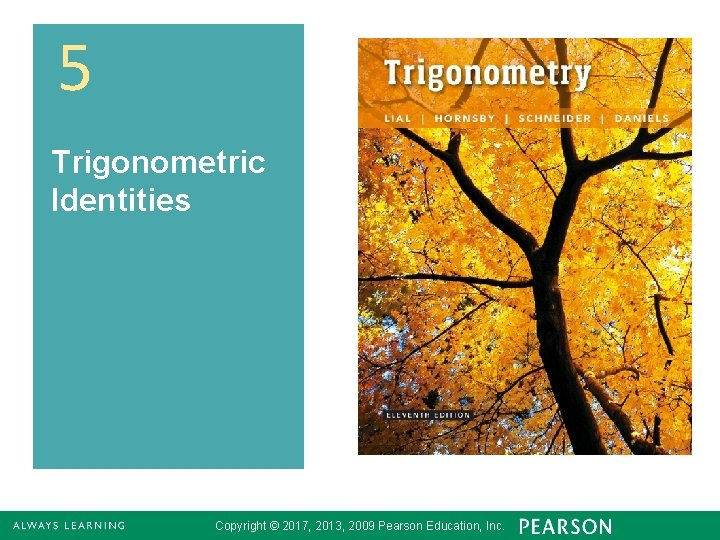5 Trigonometric Identities Copyright © 2017, 2013, 2009 Pearson Education, Inc. 1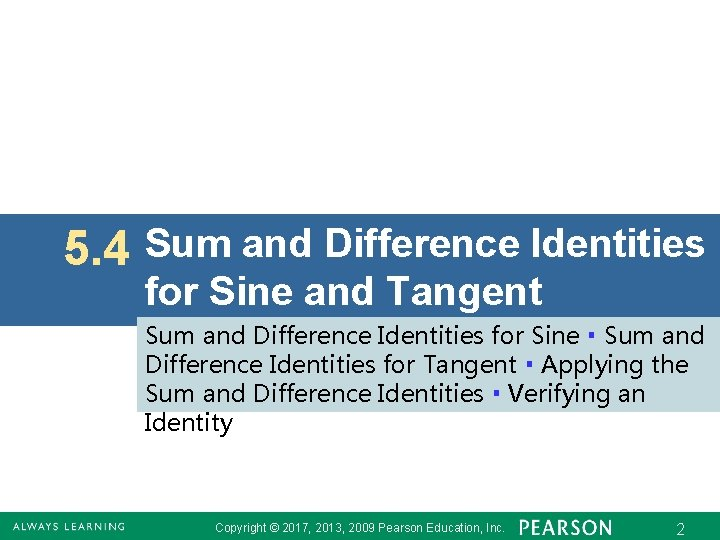5. 4 Sum and Difference Identities for Sine and Tangent Sum and Difference Identities for Sine ▪ Sum and Difference Identities for Tangent ▪ Applying the Sum and Difference Identities ▪ Verifying an Identity Copyright © 2017, 2013, 2009 Pearson Education, Inc. 2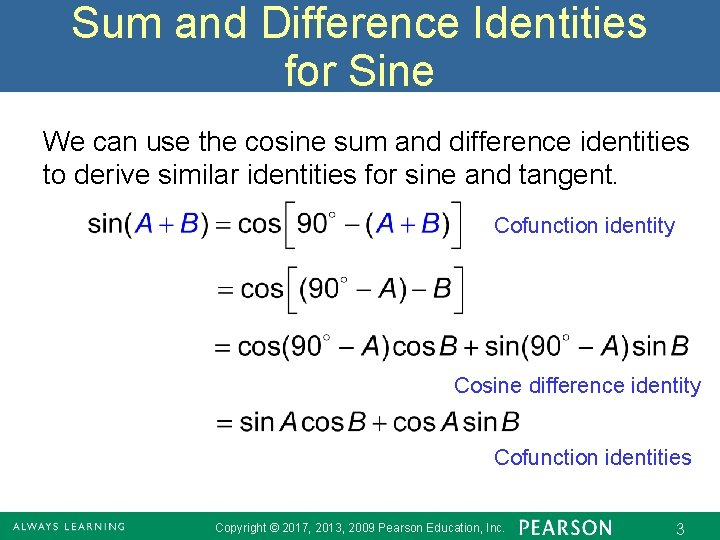Sum and Difference Identities for Sine We can use the cosine sum and difference identities to derive similar identities for sine and tangent. Cofunction identity Cosine difference identity Cofunction identities Copyright © 2017, 2013, 2009 Pearson Education, Inc. 3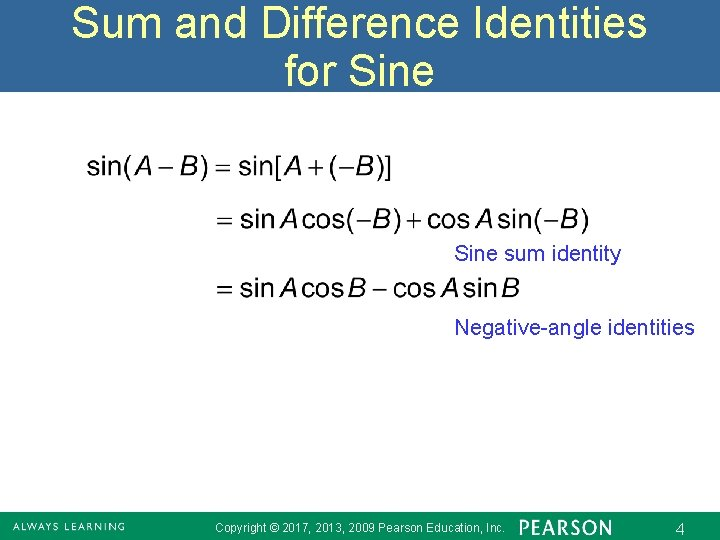Sum and Difference Identities for Sine sum identity Negative-angle identities Copyright © 2017, 2013, 2009 Pearson Education, Inc. 4Sine of a Sum or Difference Copyright © 2017, 2013, 2009 Pearson Education, Inc. 5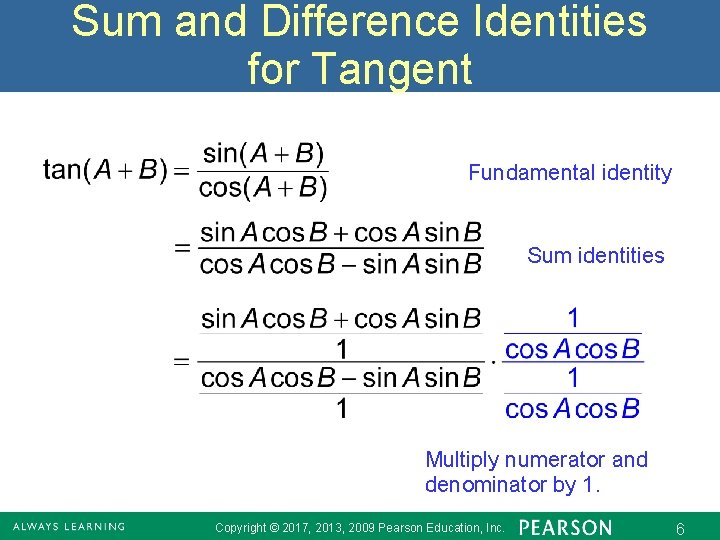Sum and Difference Identities for Tangent Fundamental identity Sum identities Multiply numerator and denominator by 1. Copyright © 2017, 2013, 2009 Pearson Education, Inc. 6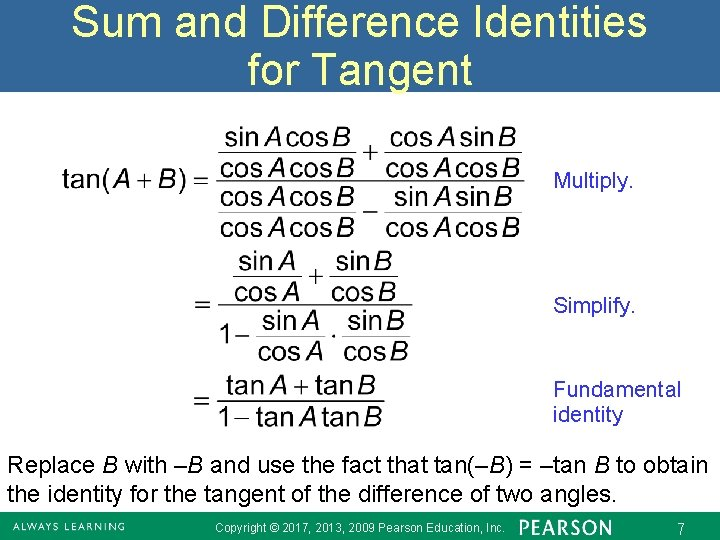Sum and Difference Identities for Tangent Multiply. Simplify. Fundamental identity Replace B with –B and use the fact that tan(–B) = –tan B to obtain the identity for the tangent of the difference of two angles. Copyright © 2017, 2013, 2009 Pearson Education, Inc. 7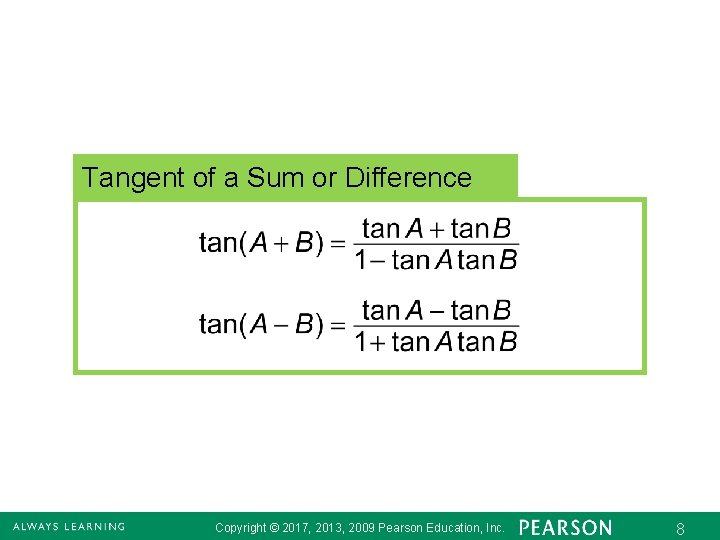Tangent of a Sum or Difference Copyright © 2017, 2013, 2009 Pearson Education, Inc. 8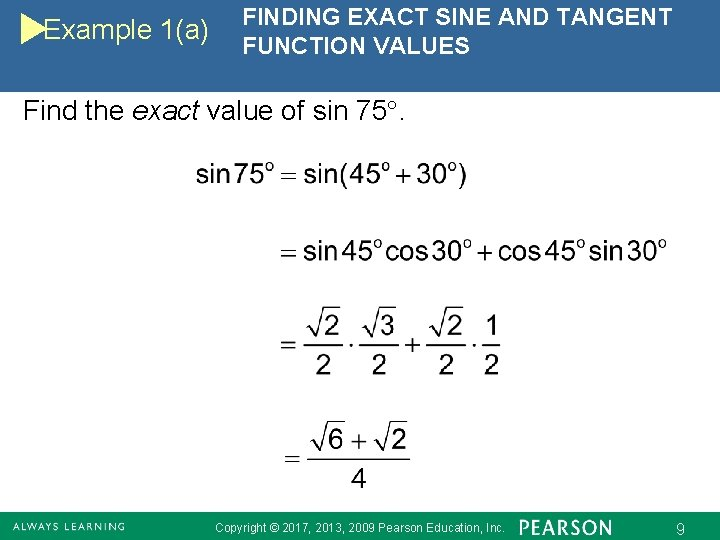Example 1(a) FINDING EXACT SINE AND TANGENT FUNCTION VALUES Find the exact value of sin 75. Copyright © 2017, 2013, 2009 Pearson Education, Inc. 9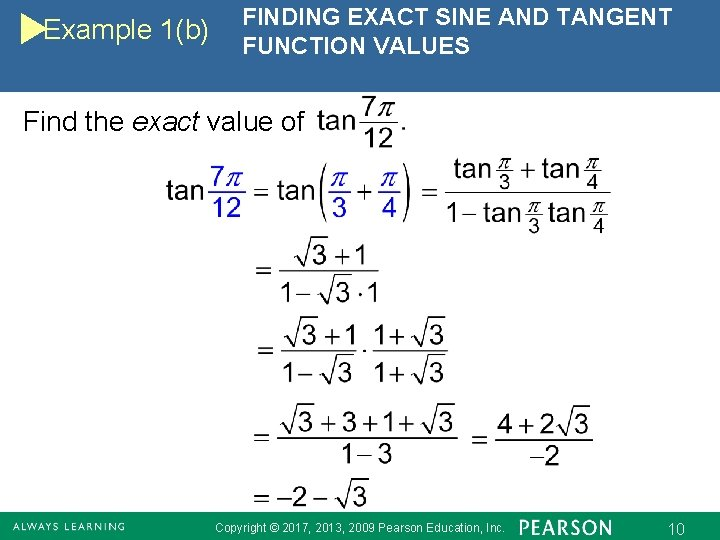Example 1(b) FINDING EXACT SINE AND TANGENT FUNCTION VALUES Find the exact value of Copyright © 2017, 2013, 2009 Pearson Education, Inc. 10Example 1(c) FINDING EXACT SINE AND TANGENT FUNCTION VALUES Find the exact value of Copyright © 2017, 2013, 2009 Pearson Education, Inc. 11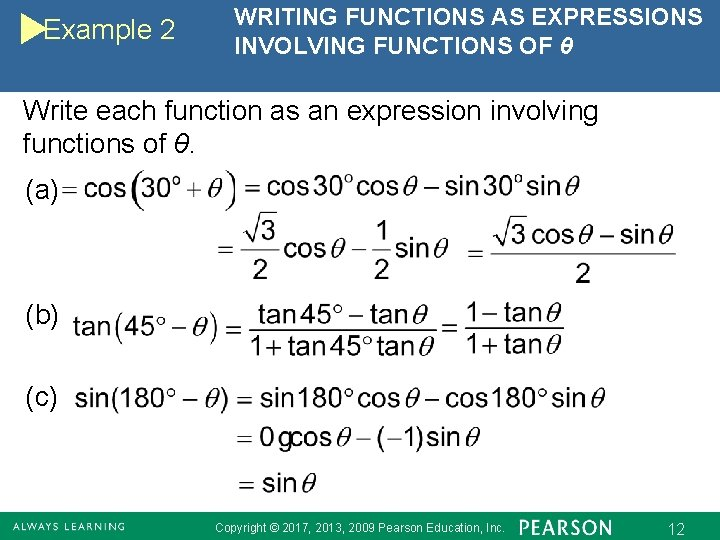Example 2 WRITING FUNCTIONS AS EXPRESSIONS INVOLVING FUNCTIONS OF θ Write each function as an expression involving functions of θ. (a) (b) (c) Copyright © 2017, 2013, 2009 Pearson Education, Inc. 12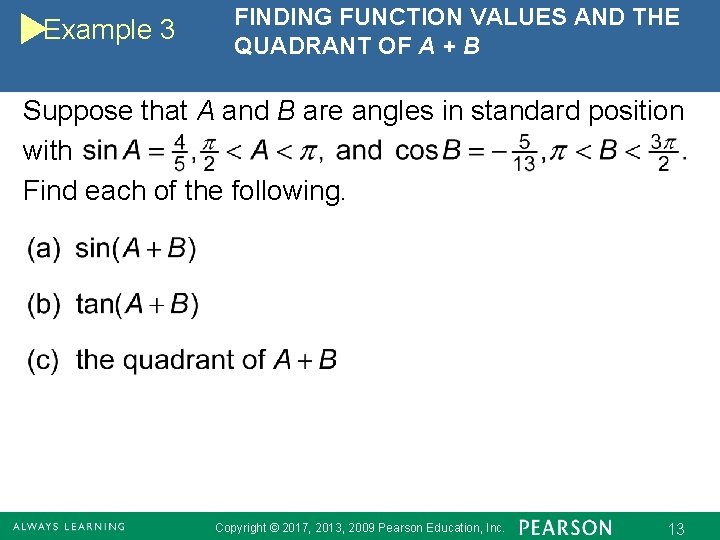Example 3 FINDING FUNCTION VALUES AND THE QUADRANT OF A + B Suppose that A and B are angles in standard position with Find each of the following. Copyright © 2017, 2013, 2009 Pearson Education, Inc. 13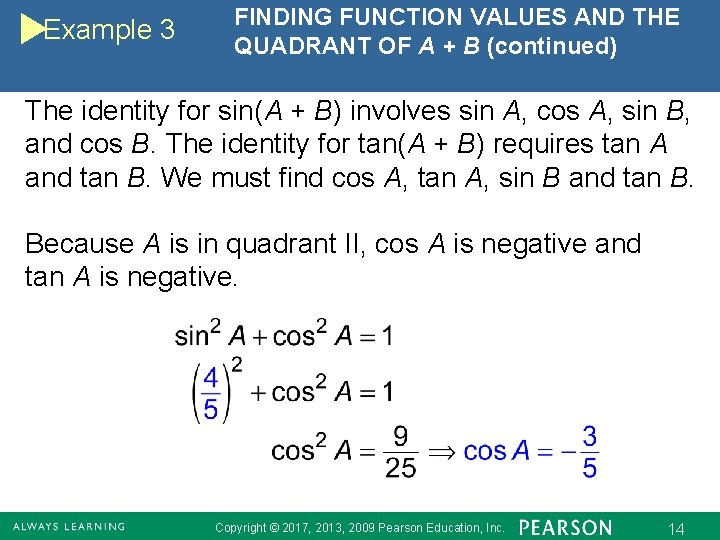Example 3 FINDING FUNCTION VALUES AND THE QUADRANT OF A + B (continued) The identity for sin(A + B) involves sin A, cos A, sin B, and cos B. The identity for tan(A + B) requires tan A and tan B. We must find cos A, tan A, sin B and tan B. Because A is in quadrant II, cos A is negative and tan A is negative. Copyright © 2017, 2013, 2009 Pearson Education, Inc. 14Example 3 FINDING FUNCTION VALUES AND THE QUADRANT OF A + B (continued) Because B is in quadrant III, sin B is negative and tan B is positive. Copyright © 2017, 2013, 2009 Pearson Education, Inc. 15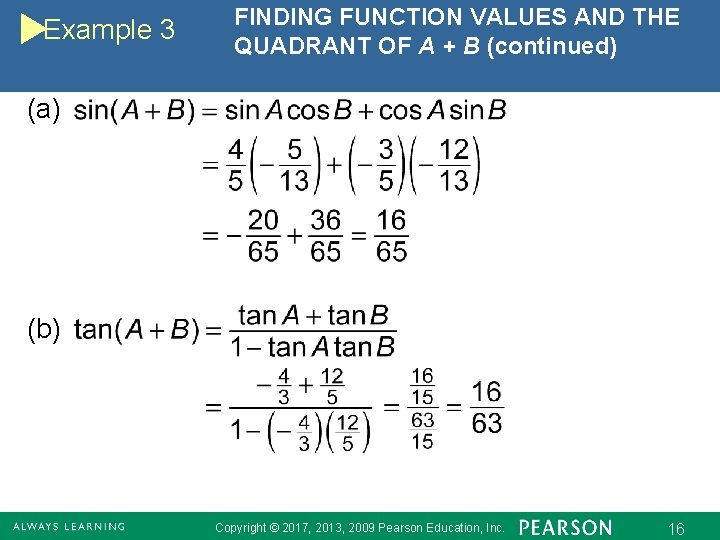Example 3 FINDING FUNCTION VALUES AND THE QUADRANT OF A + B (continued) (a) (b) Copyright © 2017, 2013, 2009 Pearson Education, Inc. 16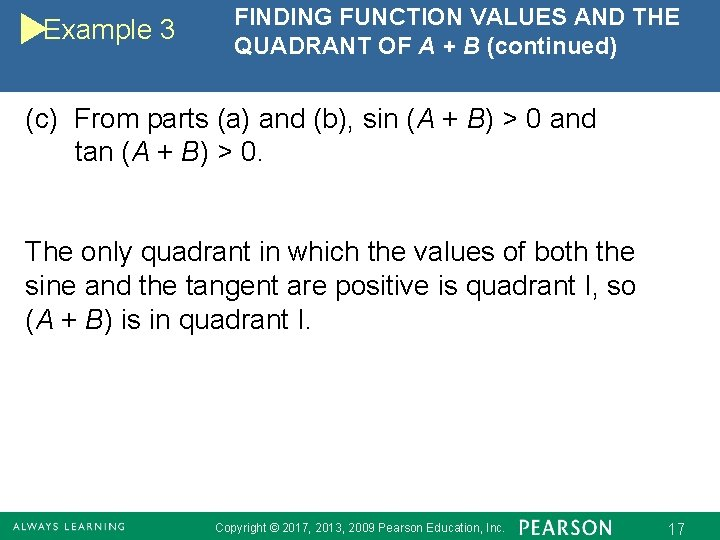Example 3 FINDING FUNCTION VALUES AND THE QUADRANT OF A + B (continued) (c) From parts (a) and (b), sin (A + B) > 0 and tan (A + B) > 0. The only quadrant in which the values of both the sine and the tangent are positive is quadrant I, so (A + B) is in quadrant I. Copyright © 2017, 2013, 2009 Pearson Education, Inc. 17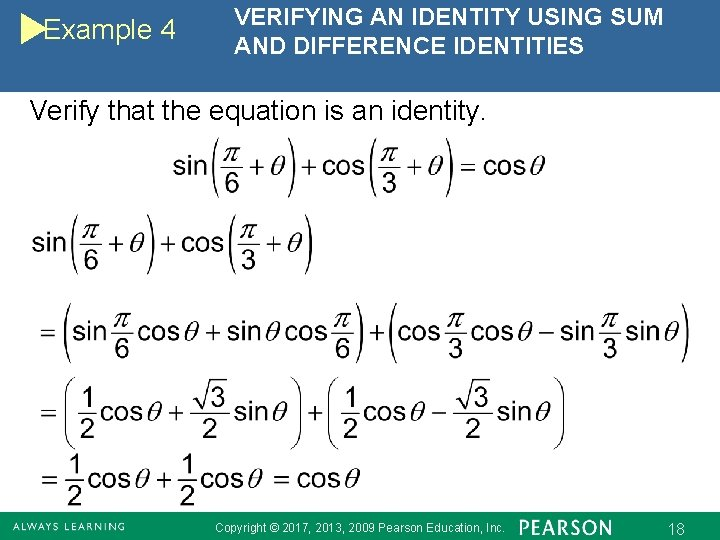Example 4 VERIFYING AN IDENTITY USING SUM AND DIFFERENCE IDENTITIES Verify that the equation is an identity. Copyright © 2017, 2013, 2009 Pearson Education, Inc. 18